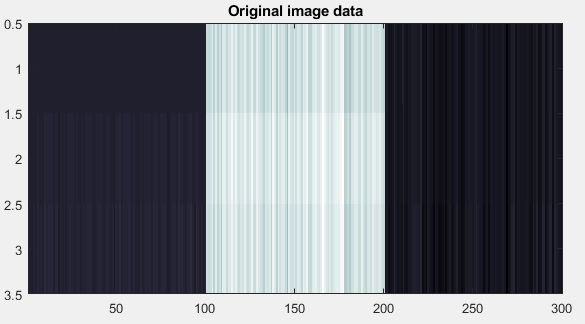Sale!

# MATLAB code of Kernel-Linear Discriminant Analysis

19

## Description

One of the methods that has been used widely for the separation of data is mapping to higher dimensional.

In this MATLAB project, Kernel-Linear Discriminant Analysis (KLD) is implemented in MATLAB.

This implementation uses the Gaussian kernel.

There is an example file that you learn how to use the functions of this MATLAB project.

Result :original imageDistance matrix original imageData after Kernel Linear Discriminant Analysisdistance matrix of the dimensionality reduced data using KLDA

## Reviews

There are no reviews yet.

SKU: KLD25BKO Category: